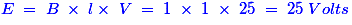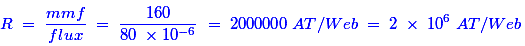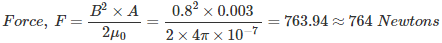# MCQs on Electromagnetic Fields

##### Page 6 of 20. Go to page 1 2 3 4 5 6 7 8 9 10 11 12 13 14 15 16 17 18 19 20
01․ The tubes of force within the magnetic material are known as
lines of force.
tubes of indication.
magnetic flux.
none of them.

If we keep one magnetic material near a magnetic flux which is produced by a separate excitation source, then the magnetic lines of force are induced in it. The groups of limited number of magnetic lines of force forming a tube like structure are referred as tubes of force by Faraday. Hence, the tubes of force within the magnetic material are known as magnetic flux.

02․ The magnetic vector potential due to a single conductor carrying current is
zero.
infinite.
finite.
none of tha above.

In a single conductor, the direction of the current is the same direction as that of magnetic vector potential. Then that can be treated as a current distribution on the surface of an infinite wire length. Hence, the magnetic vector potential is also infinite due to the single conductor carrying current.

03․ The Biot-savarts law is a general modification of
Kirchhoffs law.
Lenzs law.
Amperes law.

Because, Biot-Savart’s law describes the three different types of currents, which is nothing but the filament or line current, surface current and volume current. Hence, this law is a general modification of Ampere’s circuital law.

04․ How does the magnetic compass needle behave in a magnetic field?
It assures a position right angle to magnetic field.
It starts rotating.
It assures a position which follows a line of magnetic flux.
None of above.

The magnetic field acts upon the magnetic material of the compass needle that causes the needle to align with respect to the field.

05․ A conductor of length 1 metre moves at right angles to magnetic field of flux density 1 Wb/m2 with a velocity of 25 m/s. The induced emf in the conductor will be
25 V.
50 V.
75 V.
100 V.

Given data: Length ‘l’ = 1 meter; Flux density ‘B’ = 1 Wb/m2; Velocity ‘V’ = 25 m/s. Then, the induced in the conductor is given by the following expression.06․ A magnetic material has a total flux B of 80 micro Wb with an mmf of 160 AT. The reluctance in ampere turn per weber is
2 × 10-6.
2 × 106.
2 × 10-8.
2 × 108.

Given data: Total flux ‘Φ’ = 80 micro Weber; mmf = 160 AT; Reluctance = ? The reluctance can be calculated by the given formula.07․ The force required to seperate two surfaces with a contact area measuring 5 cm by 6 cm, when flux density normal to the surface is 0.8 tesla, will be
76400 N.
7640 N.
764 N.
76.4 N.

Given data: Area ‘A’ = (5 × 6 × 10-4) = 0.003; Flux density ‘B’ = 0.8 Tesla; Force = ? The force can be calculated by using the below expression.08․ The lagging of flux density behind the applied magnetising force is known as
permeance.
flux.
hysteresis.
all of the above

The lag or delay of a magnetic flux density known commonly as magnetic Hysteresis. This relates to the magnetization properties of a material by which it firstly becomes magnetized and then de-magnetized.

09․ The attraction capacity of electromagnet will increase if the
core length increases.
core area increases.
flux density increases.
flux density decreases.

The amount of flux production is the only one property to decide the attraction capacity of electromagnet. So, if the flux density increases, then the attraction capacity of an electromagnet will also increase.

10․ Which of following is not a unit of flux?
Maxwell.
Telsa.
Weber.
All of above.

A Weber (Wb) is a derived SI unit of magnetic flux. Maxwell is CGS unit of magnetic flux. Tesla is a SI unit of magnetic flux density or magnetic field strength. Tesla is the unit of flux density.

<<<45678>>>